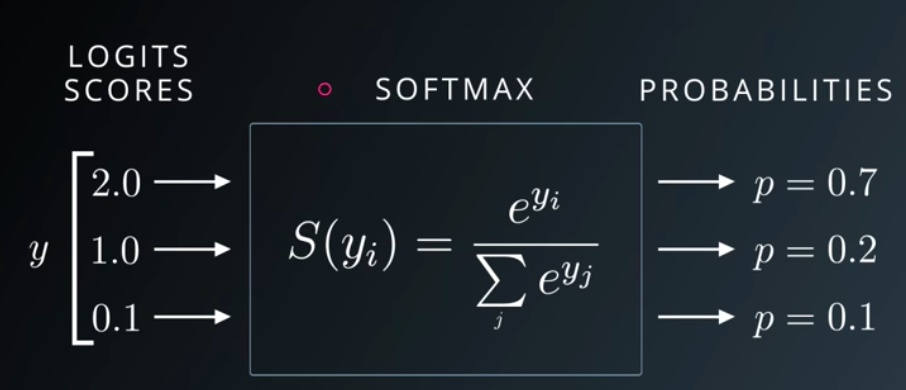# Understand the Softmax Function in Minutes

Learning machine learning? Specifically trying out neural networks and getting on the hype of deep learning? You likely have run into the softmax function, a wonderful function that turn numbers, aka logits, into probabilities that sum to one.

We will help you understand the softmax function by showing you exactly how it works — by coding your very own softmax function in python.

The above Udacity lecture slide shows that softmax function turns [2.0, 1.0, 0.1] into [0.7, 0.2, 0.1] and the probabilities sum to 1.

The softmax is not a black box. It has two components: special number e to some power divide by a sum of some sort.

yi refers to each element in y the logits vector. Let’s see it in code

`logits = [2.0, 1.0, 0.1]import numpy as npexps = [np.exp(i) for i in logits]`

We use numpy.exp(power) to take the special number e to any power we want. We use python list comprehension to iterate through each of the logits, and compute np.exp(logit). Notice the use of plural and singular form. The result is stored in a list called exps.

We just computed the top part of the softmax for each logit. Each of these transformed logit needs to be normalized by another number — the sum of all the transformed logits. This normalized give us nice probabilities outputs that sum to one!

We compute the sum of all the transformed logits and store the sum in sum_of_exps.

`sum_of_exps = sum(exps)`

Now we are ready to write the final part of our softmax function: each transformed logits need to be normalized

`softmax = [j/sum_of_exps for j in exps]`

Again, we use python list comprehension: we grab each transformed logit using [j for j in exps] divide each j by the sum_of_exps.

List comprehension gives us a list back. When we print the list we get

`>>> softmax[0.6590011388859679, 0.2424329707047139, 0.09856589040931818]>>> sum(softmax)1.0`

The output rounds to [0.7, 0.2, 0.1] as seen on the slide at the beginning of this article. They sum nicely to one!

If we hardcore our label data to the vectors below

`[1,0,0] #cat[0,1,0] #dog[0,0,1] #bird`

The output probabilities are saying 70% sure it is a cat, 20% a dog, 10% a bird. One can see that the initial differences are adjusted to percentages. logits = [2.0, 1.0, 0.1]. It’s not 2:1:0.1. Previously, we cannot say that it’s 2x more likely to be a cat, because the results were not normalized to sum to one.

Why do we still need fancy machine learning libraries with fancy softmax function? The nature of machine learning training requires ten of thousands of training data. Something as concise as the softmax function needs to be optimize to process each element. Some say that Tensorflow broadcasting is not necessarily faster than numpy’s matrix broadcasting though.Source: Deep Learning on Medium